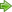﻿ Unreal Software - Thread: Predict jump height in a certain time

# ForumOff TopicPredict jump height in a certain time

#Predict jump height in a certain time

2 replies
Goto Page1User
OfflineHi, Can somebody help me with this, It's quite a complicated thing. My friend somehow managed to do this, however he hasn't told me all the solution for that. Let's assume, we are in a 3D shooter game, here's what I'm trying to do: If a player jumps for example in CSGO, ex: in 0.5 or in 2 second seconds where they will be at? the Y position? how to do the calculations for this. the formulas?what i've done so far:
I've managed to create a system to detect the maximum jump height, how long a jump would take, and whether the player is currently jumping or falling from the jump.

Presumably, the math would at least need to take into account the gravity, the jump power/speed, position, and height of the jump of the player?

Code:
1
2
3
4
5
6
7
8
9
10
11
12
13
14
15
16
17
18
19
20
21
22
23
24
25
26
27
28
29
30
31
32
33
34
35
36
37
38
39
40
41
42
43
44
45
46
local RunService = game:GetService("RunService")
local desiredInterval = 6.37 -- The time that a jump takes
local counter = 0

local isFalling = false
local jumping = false

RunService.Heartbeat:Connect(function(step)
counter = counter + step  --step is time in seconds since the previous frame

if counter >= desiredInterval/2 then -- What basically this does is: whenever the player jump is ended or the jump velocity becomes minus i guess, but hasnt landed yet and just started falling
if jumping then
if not isFalling then
print("Hit Highest Point Of The Jump")
print("Now Started falling")
isFalling = true
end
end
end

if counter >= desiredInterval then -- when all the jump is ended and player hits the ground
if jumping then
jumping = false
isFalling = false
counter = counter - desiredInterval
end
end
end)

Player.StateChanged:Connect(function(old, new)
if (new == Jumping) then
jumping = true

local h = JumpSpeed^2/(2*Gravity); -- Predicted max height of the jump
local t = 2*JumpSpeed/Gravity; -- Predicted time for the jump to take/end

desiredInterval = t
counter = 0

print("Player jumped")

print(string.format("Jump will take %s seconds and will reach a max height of %s studs", t, h));
elseif (old == Falling and new == Landed) then
print("LANDED or hit ground")
end
end)

The function I want to be filled:
Code:
1
2
3
4
5
6
7
8
9
local function PredictJumpHeightInInterval(player, interval) -- i don't know if even the player argument is necessary
local Gravity -- We have those declared already, and we do have the value.
local JumpPower -- JumpSpeed -- same as above

local h = JumpSpeed^2/(2*Gravity); -- Predicted max height of the jump
local t = 2*JumpSpeed/Gravity; -- Predicted time for the jump to take/end

-- Here code
end

Would appreciateCebra
User
OfflineI am not sure about what exactly you want to achieve, but from physics-aspect you can derive a simple equation.

Your jump height h(t) is depending on the initial height h0, the initial velocity v and the acceleration a.

h(t) = 0.5 * a * t² + v *t + h0

Your jumppower is proportional to the initial velocity and the gravity to the acceleration.

Maybe this will help?
Offline@Cebra: Thank you, after making some little changes, finally could made it work1